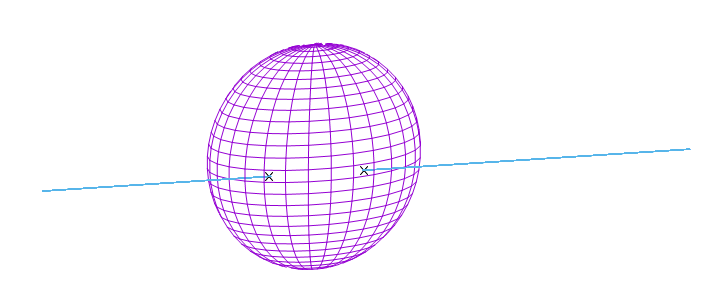## Ray-Sphere IntersectionWhen developing atmospheric scattering shaders, we will need to calculate intersection points between rays and spheres. So here is how we can do that.

Defining a ray
To define a ray, we specify two vectors: One point o, which goes from zero to a point on the ray and another one d, which goes along the ray and defines therefore the ray’s direction. The direction vector is multiplied by an argument t. When varying t, we can reach any point on the ray. Now you might say, that we did not define a ray, but a line. The only difference between rays and lines is, that t has a minimum value for rays. A line goes from -infinity to infinity, while a ray has a starting point and goes to infinity from there. In our use case, that’s not that important tough.$\displaystyle\overrightarrow{x}=\overrightarrow{o}+t\overrightarrow{d}$

Defining a sphere
To define a sphere, we need to specify a center point c and a radius r. All points on a sphere are points, which have a distance to the center point equally to the sphere’s radius. So a sphere’s definition can be:$\displaystyle\|\overrightarrow{x}-\overrightarrow{c}\|=r$ If we can assume, that the center of the sphere is at the origin of our coordinate system, the equation simplifys to$\displaystyle\|\overrightarrow{x}\|=r$, so “all vectors with a certain length r”.

Intersecting both
In our use case, we will have the spheres always centered, so we can use the easier version of the sphere definition. Inserting the ray definition into the sphere definition yields:$\displaystyle\|\overrightarrow{o}+t_{\mathrm{hit}}\overrightarrow{d}\|=r$ I replaced t with$\displaystyle t_{\mathrm{hit}}$ because now it is not a varying parameter anymore. Instead it is a symbol for all values which fulfill this equation (a ray can hit a sphere zero, one or two times).
Image a vector$\displaystyle\overrightarrow{a}\$, then there is:$\displaystyle\|\overrightarrow{a}\|^2 = \|\overrightarrow{a}\|\|\overrightarrow{a}\|$

From the definition of the dot product and$\displaystyle\cos{0}=1 \Rightarrow \overrightarrow{a}\cdot\overrightarrow{a}=\|\overrightarrow{a}\|\|\overrightarrow{a}\|\cos{\alpha} = \|\overrightarrow{a}\|\|\overrightarrow{a}\|$
So there is$\displaystyle\|\overrightarrow{a}\|^2 = \overrightarrow{a}\cdot\overrightarrow{a}$

To apply this to the intersection, we need to square it first:$\displaystyle\|\overrightarrow{o}+t_{\mathrm{hit}}\overrightarrow{d}\|=r \Leftrightarrow r^2 =\|\overrightarrow{o}+t_{\mathrm{hit}}\overrightarrow{d}\|^2$$=(\overrightarrow{o}+t_{\mathrm{hit}}\overrightarrow{d})\cdot(\overrightarrow{o}+t_{\mathrm{hit}}\overrightarrow{d})$$\displaystyle=\overrightarrow{o}^2+2\overrightarrow{o}t_{\mathrm{hit}}\overrightarrow{d}+(t_{\mathrm{hit}}\overrightarrow{d})^2 =\overrightarrow{o}^2+2\overrightarrow{o}\cdot\overrightarrow{d}t_{\mathrm{hit}}+\overrightarrow{d}^2t_{\mathrm{hit}}^2$$\displaystyle\Leftrightarrow\overrightarrow{o}^2+2\overrightarrow{o}\cdot\overrightarrow{d}t_{\mathrm{hit}}+\overrightarrow{d}^2t_{\mathrm{hit}}^2-r^2=0$$\displaystyle\Leftrightarrow \overrightarrow{d}^2t_{\mathrm{hit}}^2 +2\overrightarrow{o}\cdot\overrightarrow{d}t_{\mathrm{hit}}+\overrightarrow{o}^2-r^2=0$$\displaystyle\Leftrightarrow t_{\mathrm{hit}}^2 +\frac{2\overrightarrow{o}\cdot\overrightarrow{d}}{\overrightarrow{d}^2}t_{\mathrm{hit}}+\frac{\overrightarrow{o}^2-r^2}{\overrightarrow{d}^2}=0$

To make the calculation easier we assume, that$\displaystyle\overrightarrow{d}$ is normalized:$\displaystyle\|\overrightarrow{d}\|=1 \Rightarrow \overrightarrow{d}^2 = \overrightarrow{d}\cdot\overrightarrow{d} = \|\overrightarrow{d}\|\|\overrightarrow{d}\| = 1*1 = 1$ So the denominators above are one and are left out.

Now we use the reduced quadratic formula to solve the equation for$\displaystyle t_{\mathrm{hit}}$:$\displaystyle p=2\overrightarrow{o}\overrightarrow{d}, q=\overrightarrow{o}^2-r^2$
The discriminant is$\displaystyle D=\left(\frac{p}{2}\right)^2-q$$\displaystyle t_{\mathrm{hit}}=-\frac{p}{2}\pm\sqrt{D}$$\displaystyle=-\frac{2\overrightarrow{o}\overrightarrow{d}}{2}\pm\sqrt{\left(\frac{2\overrightarrow{o}\overrightarrow{d}}{2}\right)^2-\overrightarrow{o}^2+r^2}$$\displaystyle= -\overrightarrow{o}\overrightarrow{d}\pm\sqrt{\left(\overrightarrow{o}\overrightarrow{d}\right)^2-\overrightarrow{o}^2+r^2}$
So the cheapest way to calculate the intersection point is:$\displaystyle t_{\mathrm{hit}}=-a\pm\sqrt{a^2-\overrightarrow{o}^2+r^2}$ with$a=\overrightarrow{o}\cdot\overrightarrow{d}$

Keep in mind, that$\overrightarrow{d}$ has to be normalized!